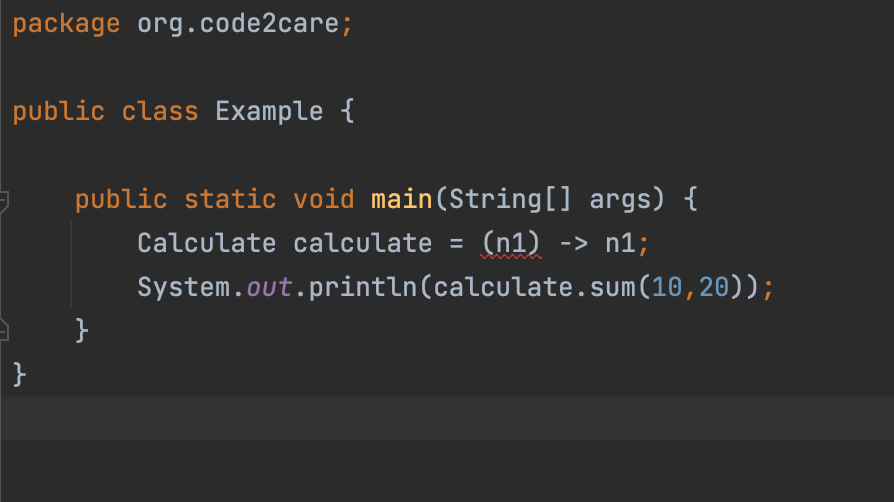# [Fix] java: incompatible types: incompatible parameter types in lambda expression error

``java: incompatible types: incompatible parameter types in lambda expression``

If you are using a Functional Interface with a method that say takes in to two arguments and when implementing the method using Lambda expression you pass in not two you will get this error. Let's see an example

Example: Functional Interface
``````package org.code2care;

@FunctionalInterface
public interface Calculate {

public int sum(int number1,int number2);

}``````
Lambda Expression:
``````package org.code2care;

public class Example {

public static void main(String[] args) {
Calculate calculate = (n1) -> n1; //Compilation error
System.out.println(calculate.sum(10,20));
}
}``````

❗️Incompatible parameter types in lambda expression: wrong number of parameters: expected 2 but found 1

So the fix to incompatible parameter types in lambda expression is to make sure that you pass in the correct parameters and types when trying to define a lambda expression.

``````    public static void main(String[] args) {
Calculate calculate = (n1,n2) -> n1+n2; //fix
System.out.println(calculate.sum(10,20));
}``````Incompatible parameter types in lambda expression: wrong number of parameters: expected 2 but found 1